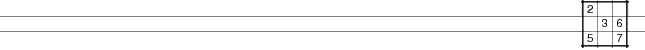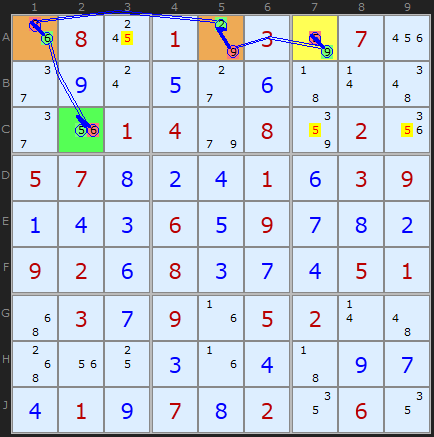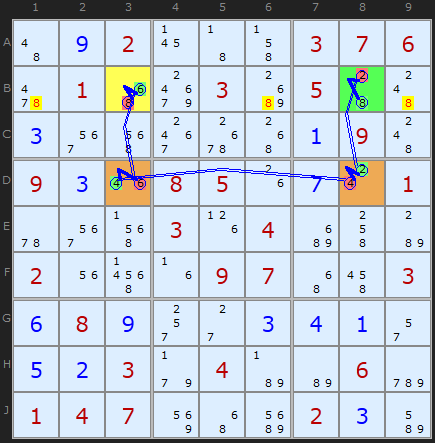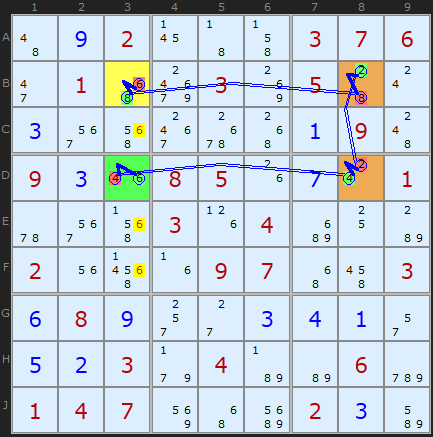OCTYPE html> SudokuWiki.org - XY-Chains, sudokuwiki.org
 Main Page - Back

# XY-Chains

From sudokuwiki.org, the puzzle solver's siteXY-Chain example 1 : Load Example or : From the Start The Y-Wing Chains are infact part of a more encompassing strategy called XY-Chains. The commonality is the same pincer-like attack on candidates that both ends can see and that the chain is made of bi-value cells. With Y-Chains the hinge was expanded to a chain of identical bi-value cells but in an XY-Chain these can be different - as long as there is one candidate to make all the links. The "X" and the "Y" in the name represent these two values in each chain link.

The example here is a very simple XY-Chain of length 4 which removed all 5's highlighted in yellow. The chain ends are 5 A7 and C2 - so all cells that can see both of these are under fire. It's possible to start at either end but lets follow the example from A7. We can reason as follows

• If A7 is 5 then A3/C7/C9 cannot be.
• if A7 is NOT 5 then it's 9, so A5 must be 2, which forces A1 to be 6. If A1 is 6 then C2 is 5.

Which ever choice in A7 the 5's in A3/C7/C9 cannot be 5. The same logic can be traced from C2 to A7 so the strategy is bi-directional, in the jargon.XY-Chain example 2 : Load Example or : From the Start
This next Sudoku puzzle contains an entertaining series of XY-Chains, starting with this rectangular one. It proves that 8 must be in either B3 or B8 and therefore we can remove the other three 8s in row B. Starting on B3 if that cell is either 8 or 6. If it is 6 then D3 must be 4 which pushes 2 into D8 which in turn makes B8 8. You can trace this from B8 back round for the same effect. A nice short XY-Chain, but as the next example shows, these four cells are a rich seam.Same cells - different XY-Chain : Load Example or : From the Start
Looking at exactly the same starting cell it appears we can make further eliminations, this time 6s in column 3. We go clock-wise, this time, round the rectangle. It proves 6 will either be on B3 or D3.

If you want to finish the puzzle by yourself, look out for a third elimination with those same four cells using 2s on column 8, or step through with the solver.

## XY-Chains Exemplars

These puzzles require the XY-Chains strategy at some point but are otherwise trivial.
They also require one Naked Pair (except for #5).
They make good practice puzzles. Thanks to Klaus Brenner for number 5

 Go back to Y-Wing Chains Continue to 3D Medusa